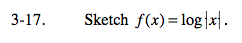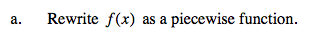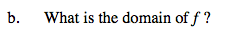### Home > CALC > Chapter 3 > Lesson 3.1.1 > Problem3-17

3-17.
1. Sketch f(x) = log|x|. Homework Help ✎

1. Rewrite f(x) as a piecewise function.

2. What is the domain of f ?This piecewise will have two pieces with a boundary point at x = 0.The domain of the parent graph, y = log(x), is x > 0. In other words, negative values and 0 are restricted.
For f(x) = log|x|, are negative values restricted? How about 0?